Next: Worked Examples Up: Electromagnetic Waves Previous: Effect of Dielectric Materials

## Energy in Electromagnetic Waves

From Sect. 233, the energy stored per unit volume in an electromagnetic wave is given by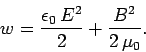(331)

Since,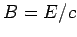, for an electromagnetic wave, and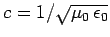, the above expression yields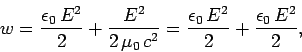(332)

or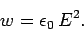(333)

It is clear, from the above, that half the energy in an electromagnetic wave is carried by the electric field, and the other half is carried by the magnetic field.

As an electromagnetic field propagates it transports energy. Let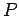be the power per unit area carried by an electromagnetic wave: i.e.,is the energy transported per unit time across a unit cross-sectional area perpendicular to the direction in which the wave is traveling. Consider a plane electromagnetic wave propagating along the-axis. The wave propagates a distance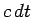along the-axis in a time interval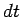. If we consider a cross-sectional areaat right-angles to the-axis, then in a timethe wave sweeps through a volume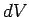of space, where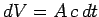. The amount of energy filling this volume is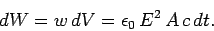(334)

It follows, from the definition of, that the power per unit area carried by the wave is given by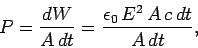(335)

so that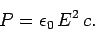(336)

Since half the energy in an electromagnetic wave is carried by the electric field, and the other half is carried by the magnetic field, it is conventional to convert the above expression into a form involving both the electric and magnetic field strengths. Since,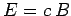, we have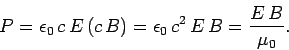(337)

Thus,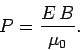(338)

Equation (338) specifies the power per unit area transported by an electromagnetic wave at any given instant of time. The peak power is given by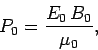(339)

where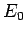and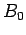are the peak amplitudes of the oscillatory electric and magnetic fields, respectively. It is easily demonstrated that the average power per unit area transported by an electromagnetic wave is half the peak power, so that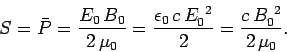(340)

The quantityis conventionally termed the intensity of the wave.Next: Worked Examples Up: Electromagnetic Waves Previous: Effect of Dielectric Materials
Richard Fitzpatrick 2007-07-14